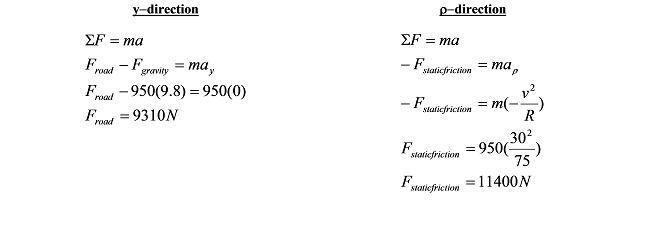# 03. Applying Newton's Second Law to Circular Motion

$$\newcommand{\vecs}{\overset { \rightharpoonup} {\mathbf{#1}} }$$ $$\newcommand{\vecd}{\overset{-\!-\!\rightharpoonup}{\vphantom{a}\smash {#1}}}$$$$\newcommand{\id}{\mathrm{id}}$$ $$\newcommand{\Span}{\mathrm{span}}$$ $$\newcommand{\kernel}{\mathrm{null}\,}$$ $$\newcommand{\range}{\mathrm{range}\,}$$ $$\newcommand{\RealPart}{\mathrm{Re}}$$ $$\newcommand{\ImaginaryPart}{\mathrm{Im}}$$ $$\newcommand{\Argument}{\mathrm{Arg}}$$ $$\newcommand{\norm}{\| #1 \|}$$ $$\newcommand{\inner}{\langle #1, #2 \rangle}$$ $$\newcommand{\Span}{\mathrm{span}}$$ $$\newcommand{\id}{\mathrm{id}}$$ $$\newcommand{\Span}{\mathrm{span}}$$ $$\newcommand{\kernel}{\mathrm{null}\,}$$ $$\newcommand{\range}{\mathrm{range}\,}$$ $$\newcommand{\RealPart}{\mathrm{Re}}$$ $$\newcommand{\ImaginaryPart}{\mathrm{Im}}$$ $$\newcommand{\Argument}{\mathrm{Arg}}$$ $$\newcommand{\norm}{\| #1 \|}$$ $$\newcommand{\inner}{\langle #1, #2 \rangle}$$ $$\newcommand{\Span}{\mathrm{span}}$$$$\newcommand{\AA}{\unicode[.8,0]{x212B}}$$

# Applying Newton's Second Law to Circular Motion

Investigate the situation below, in which an object travels on a circular path.

During a high speed auto chase in San Francisco, a 1745 kg police cruiser traveling at constant speed loses contact with the road as it goes over the crest of a hill with radius of curvature 80 m.

A free-body diagram for the car at the instant it’s at the crest of the hill is sketched below.Since the car is traveling along a circular path, it’s best to analyze the situation using polar coordinates. Remember, in polar coordinates the radial direction points away from the center of the circular path.

In general, you should apply Newton’s second law independently in the radial (r) and tangential (f) directions, although since the cruiser is moving at constant speed, there is no acceleration in the tangential direction and therefore no net force in the tangential direction.Since the cruiser loses contact with the road, Froad = 0 N.

$0 - 1745(9.8) = -1745(80) \omega^2$

$\omega = 0.35 \; rad/s$

Since $$v = R \omega$$,

$v = (80 m)(0.35 \; rad/s)$

$v =28 \; m/s$

For the car to lose contact with the road, it must be traveling at a speed of 28 m/s or greater when it reaches the crest of the hill. At lower speeds, the car would remain contact with the road.

Since it is often useful to directly relate the radial acceleration of an object to its velocity, let's construct a direct relationship between these two variables:

$a_\rho =R \omega ^2$

$a_\rho = -R \left( \frac{v}{R} \right )^2$

$a_\rho = \frac{-v^2}{R}$

This relationship, although mathematically equivalent to $$a_\rho =R \omega ^2$$, is often a more "useful" form.

# Another Circular Motion Scenario

A 950 kg car, traveling at a constant 30 m/s, safely makes a lefthand-turn with radius of curvature 75 m.

First, let's draw a pair of free-body diagrams for the car, a side-view (on the left) and a rear-view (on the right)The free-body diagram on the left is a side-view of the car. Notice that the upward direction is the y-direction, and the forward direction, tangent to the turn, is the f-direction.

The free-body diagram on the right is a rear-view of the car. This is what you would see if you stood directly behind the car. Notice that the upward direction is still the y-direction, and the horizontal direction, perpendicular to the direction of travel and hence directed radially outward, is the r-direction. The frictional force indicated is perpendicular to the tread on the tire. This force causes the car to accelerate toward the center of the turn. Remember, if the car is going to travel along a circular path, it must have an acceleration directed toward the center of the circle. Something has to be supplying the force that creates this acceleration. This something is the static friction between the tire and the road that acts to prevent the car from sliding out of the turn. Since the car has no velocity in the radial direction, the frictional force the points in this direction must be static!

Now that we have all that straightened out (maybe), let's apply Newton's Second Law.Thus, to safely make this turn requires at least 1140 N of static friction. Using this value, I should be able to compute the minimum coefficient of friction necessary for the car to safely round this turn at this speed.

$F_{stiction} \le \mu_sF_{road}$

$11400 \le \mu_s(9310)$

$\mu_s \ge 1.22$

Although this is a large value for the coefficient of static friction, it is an attainable value for a sports car with performance tires.

This page titled 03. Applying Newton's Second Law to Circular Motion is shared under a CC BY-NC-SA license and was authored, remixed, and/or curated by Paul D'Alessandris.